# Vector Math Tutorial

2014-01-22

This tutorial will teach you the basics of vector math. Vectors are useful in 2D and 3D graphics, collision detection, physics, and many other areas of game programming.

## DefinitionA vector is a set of two or more numbers, often used to indicate a spatial position or a direction. In contrast, a scalar is a value consisting of a single numeric component; each component of a vector is a scalar. A two-component vector can be used for two-dimensional space, a three-component vector can be used for three-dimensional space, and so on. The following structs will be used throughout this tutorial to represent vectors:

```typedef struct Vector2f { float x; float y; } Vector2f; typedef struct Vector3f { float x; float y; float z; } Vector3f; ```

This tutorial uses a right-handed coordinate system where the x axis goes from left to right, the y axis goes from bottom to top, and the z axis goes from far to near. This corresponds to the coordinate system most commonly used for three-dimensional OpenGL projections.

## Position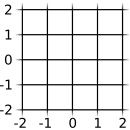When a vector is used to represent a spatial position, each component is an offset in euclidean space on the axis corresponding to it. The numeric units could be pixels, inches, meters, or anything else; it depends on the coordinate system you use. The origin in a coordinate system is the position represented by the vector {0, 0, 0}; world coordinates are measured relative to the origin. You might also sometimes use local coordinates, which are measured relative to a position that could be different from the origin.

## Direction

A vector can also be used to represent a direction. A direction vector is no different from a vector used to represent a position - the only difference is in how you interpret it. Direction vectors always exist in a local coordinate system; their direction is represented by the offset of their values relative to {0, 0, 0}.

## MagnitudeThe magnitude of a vector, also known as its length, is its distance from the origin. Vectors of equal magnitude can be visualized as points on the outside of a sphere (3D) or a circle (2D) with a radius equal to the vector's magnitude. You can normalize a vector, making its magnitude equal to 1. This is useful for a number of things. Example: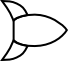Let's say you're writing a game in which the player flies a spaceship. You want to be able to move the spaceship not only left, right, up, and down, but also diagonally. When you're moving right, the spaceship goes one unit along the x axis; when moving up, it goes one unit along the y axis. But what if you're moving both right and up at the same time?To move diagonally, you can't simply move one unit to the right and one unit up, since that's a longer distance (approximately 1.4 times as far) than moving one unit on only one axis. And what if you want to move the spaceship at a steeper or shallower angle than 45 degrees?This problem can be easily solved by using a normalized direction vector. The vector points in the direction the spaceship is facing; when you move the spaceship, you use the vector to calculate the change in position. For example:

```#define SPACESHIP_MOVE_SPEED 0.2f // Arbitrary value; distance the spaceship moves per frame spaceship.position.x += SPACESHIP_MOVE_SPEED * spaceship.direction.x; spaceship.position.y += SPACESHIP_MOVE_SPEED * spaceship.direction.y; spaceship.position.z += SPACESHIP_MOVE_SPEED * spaceship.direction.z; ```

Using a normalized vector, the spaceship moves the same distance regardless of which way it's facing. This certainly isn't the only way; you could accomplish the same thing by storing the ship's direction as an angle, and using the trigonometric functions `sin` and `cos`.

## Construction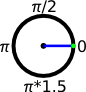There are many different ways to construct a vector. The simplest way is to assign each component directly. In the coordinate system we're using, the vector {1, 0, 0} points straight to the right; {-1, 0, 0} points straight to the left; {0, 1, 0} points straight up, etc. For values that don't point directly up or down one axis, you may want to compute them using the `cos` and `sin` functions. These functions, given an angle in radians, will return numbers you can use to construct a normalized direction vector. This only works for two out of three axes, though; the third must be set to zero.

```float angle = M_PI / 4.0f; // 45 degrees vector.x = cos(angle); vector.y = sin(angle); vector.z = 0.0f; ```

If you want to construct a vector in local coordinates (from point A, how far and in what direction is point B?), you can do it by subtracting each component in point B from the corresponding component in point A. (If you only want the direction, and not the distance, you'll need to normalize the vector afterward):

```vector.x = pointB.x - pointA.x; vector.y = pointB.y - pointA.y; vector.z = pointB.z - pointA.z; ```

## Normalization

A normalized vector has a magnitude of 1. Normalized vectors pointing in any direction are of equal distance from the origin, as though constrained to an imaginary sphere or circle. Vector normalization is done like this:

```void Vector2f_normalize(Vector2f * vector) { float magnitude; magnitude = sqrt(vector->x * vector->x + vector->y * vector->y); vector->x /= magnitude; vector->y /= magnitude; } void Vector3f_normalize(Vector3f * vector) { float magnitude; magnitude = sqrt(vector->x * vector->x + vector->y * vector->y + vector->z * vector->z); vector->x /= magnitude; vector->y /= magnitude; vector->z /= magnitude; } ```

What are we doing here? First, we use the distance formula to calculate the length, or magnitude, of the vector. For a vector that's already normalized, this will be approximately equal to 1. ("Approximately" because floating point numbers have limited precision, and rounding errors can cause things not to add up exactly.) Once we have the magnitude, we divide each component of the vector by it.

Note that if you attempt to normalize a vector with a magnitude of zero, you'll end up with a vector full of NaNs. (NaN stands for "Not a Number"; it's a special value returned from illegal operations such as a divide by zero. Languages other than C may behave differently.)

## Rotation

Rotating a vector can be done a few different ways. Three-dimensional rotation can be accomplished by multiplying the vector by a quaternion or a rotation matrix; see Quaternion Math and Matrix Math for more details. Two-dimensional rotation can be accomplished using `sin` and `cos` like this:

```#define SHIP_ROTATION_SPEED (M_PI / 100.0) void rotateShip(Spaceship * ship, float radians) { Vector2f result; result.x = ship->direction.x * cos(radians) + ship->direction.y * -sin(radians); result.y = ship->direction.x * sin(radians) + ship->direction.y * cos(radians); ship->direction = result; } void leftArrowKey(Spaceship * ship) { rotateShip(ship, -SHIP_ROTATION_SPEED); } void rightArrowKey(Spaceship * ship) { rotateShip(ship, SHIP_ROTATION_SPEED); } ```First we define the constant `SHIP_ROTATION_SPEED`, which is an arbitrary value of radians; the higher the number, the more quickly the ship will rotate. When the left arrow key is pressed, it calls `rotateShip` with a negative `radians` value, which rotates the ship counter-clockwise. When the right arrow key is pressed, it calls `rotateShip` with a positive `radians` value, which rotates the ship clockwise.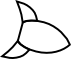`rotateShip` constructs a temporary vector to store the result of rotation. This is necessary because both the x and y components of the original vector are used in computing each resulting component; if we modified x in place, the wrong value would be used to compute the new y. `sin` and `cos` are used in a simple 2x2 matrix multiply which performs the rotation.

Here's a less efficient, but possibly easier to understand implementation using `atan2`. The `atan2` function can be used to do the inverse of `sin` and `cos`; that is, it takes coordinates as arguments and returns an angle in radians:

```void rotateShip(Spaceship * ship, float radians) { float angle; angle = atan2(ship->direction.y, ship->direction.x); angle += radians; ship->direction.x = cos(angle); ship->direction.y = sin(angle); } ```

## Dot product

Dot product is a vector operation that can be used to compute the angle between two vectors:

```float Vector2f_dot(Vector2f vector1, Vector2f vector2) { return vector1.x * vector2.x + vector1.y * vector2.y; } float Vector3f_dot(Vector3f vector1, Vector3f vector2) { return vector1.x * vector2.x + vector1.y * vector2.y + vector1.z * vector2.z; } ```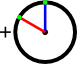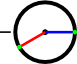The dot product has some interesting properties. If you take the dot product of two normalized vectors, you can get the angle between the vectors using `acos(dotProduct)`. (The `acos` function does the inverse of the `cos` function; it takes an x coordinate and returns an angle.)

Since the dot product is the cosine of the angle between two normalized vectors, you can also use it to determine how similar they are to each other. If the vectors are at a right angle to each other, their dot product is equal to 0. If the angle between them is less than 90°, the dot product is positive. If the angle is greater than 90°, the dot product is negative. If the vectors are the same, the dot product is equal to the square of the vectors' magnitude.

## Cross product

Cross product is a vector operation that can be used to compute a value perpendicular to two vectors:

```Vector3f Vector3f_cross(Vector3f vector1, Vector3f vector2) { Vector3f result; result.x = vector1.y * vector2.z - vector1.z * vector2.y; result.y = vector1.z * vector2.x - vector1.x * vector2.z; result.z = vector1.x * vector2.y - vector1.y * vector2.x; return result; } ```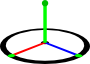There are a few catches to using cross product. First, the result will not be of the same magnitude as `vector1` and `vector2` unless they are both normalized and at an exact right angle to each other. Second, if you take the cross product of two parallel vectors, the result will have a magnitude of 0. Third, cross product is not commutative - `Vector3f_cross(a, b)` gives a result opposite to the vector returned from `Vector3f_cross(b, a)`.

In three dimensions, cross product is a vector, but in two dimensions it's a scalar:

```float Vector2f_cross(Vector2f vector1, Vector2f vector2) { return vector1.x * vector2.y - vector1.y * vector2.x; } ```

Keen observers will note that this is the same equation used for the z axis of a three dimensional cross product. Some properties of scalar cross product: If the result of `Vector2f_cross(a, b)` is positive, the shortest rotation from a to b is clockwise; if it's negative, the shortest rotation is counterclockwise. One possible use of this property is to determine whether two line segments intersect:

```// Points 0 and 1 are the endpoints of one line; 2 and 3 are the endpoints of the other bool linesIntersect(Vector2f point0, Vector2f point1, Vector2f point2, Vector2f point3) { // Subtraction pseudocode used for brevity; a - b = (Vector2f) {a.x - b.x, a.y - b.y} return signbit(Vector2f_cross(point1 - point0, point2 - point0)) != signbit(Vector2f_cross(point1 - point0, point3 - point0)) && signbit(Vector2f_cross(point3 - point2, point0 - point2)) != signbit(Vector2f_cross(point3 - point2, point1 - point2)); } ```

## Normal

A normal vector is a type of direction vector which represents the orientation of a plane. The normal of a plane points outward perpendicular to the front surface of it. For example, the floor of a room would have a normal vector of {0, 1, 0}. Normals are used in vector reflection and projection.

## Reflection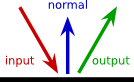Given a direction vector and a plane normal, reflecting the vector reverses it as if the plane were a mirror:

```Vector2f Vector2f_reflect(Vector2f vector, Vector2f normal) { Vector2f result; float dot; dot = Vector2f_dot(vector, normal); result.x = vector.x - 2.0f * dot * normal.x; result.y = vector.y - 2.0f * dot * normal.y; return result; } Vector3f Vector3f_reflect(Vector3f vector, Vector3f normal) { Vector3f result; float dot; dot = Vector3f_dot(vector, normal); result.x = vector.x - 2.0f * dot * normal.x; result.y = vector.y - 2.0f * dot * normal.y; result.z = vector.z - 2.0f * dot * normal.z; return result; } ```

## ProjectionGiven a position vector and a plane normal, projecting the vector gives the closest position that lies on that plane:

```Vector2f Vector2f_project(Vector2f vector, Vector2f normal) { float dot; dot = Vector2f_dot(vector, normal); vector.x -= normal.x * dot; vector.y -= normal.y * dot; return vector; } Vector3f Vector3f_project(Vector3f vector, Vector3f normal) { float dot; dot = Vector3f_dot(vector, normal); vector.x -= normal.x * dot; vector.y -= normal.y * dot; vector.z -= normal.z * dot; return vector; } ```